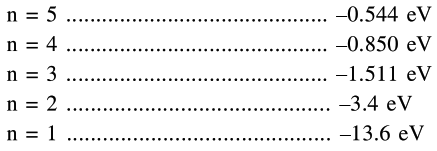# According to BohrQuestion:

According to Bohr atom model, in which of the following transitions will the frequency be maximum ?

1. $\mathrm{n}=4$ to $\mathrm{n}=3$

2. $\mathrm{n}=2$ to $\mathrm{n}=1$

3. $n=5$ to $n=4$

4. $\mathrm{n}=3$ to $\mathrm{n}=2$

Correct Option: , 2

Solution:

$\Delta \mathrm{E}=13.6\left[\frac{1}{\mathrm{n}_{1}^{2}}-\frac{1}{\mathrm{n}_{2}^{2}}\right]=\mathrm{hv}$

It is maximum if $\mathrm{n}_{1}=1$ and $\mathrm{n}_{2}=2$Option (2) is correct.